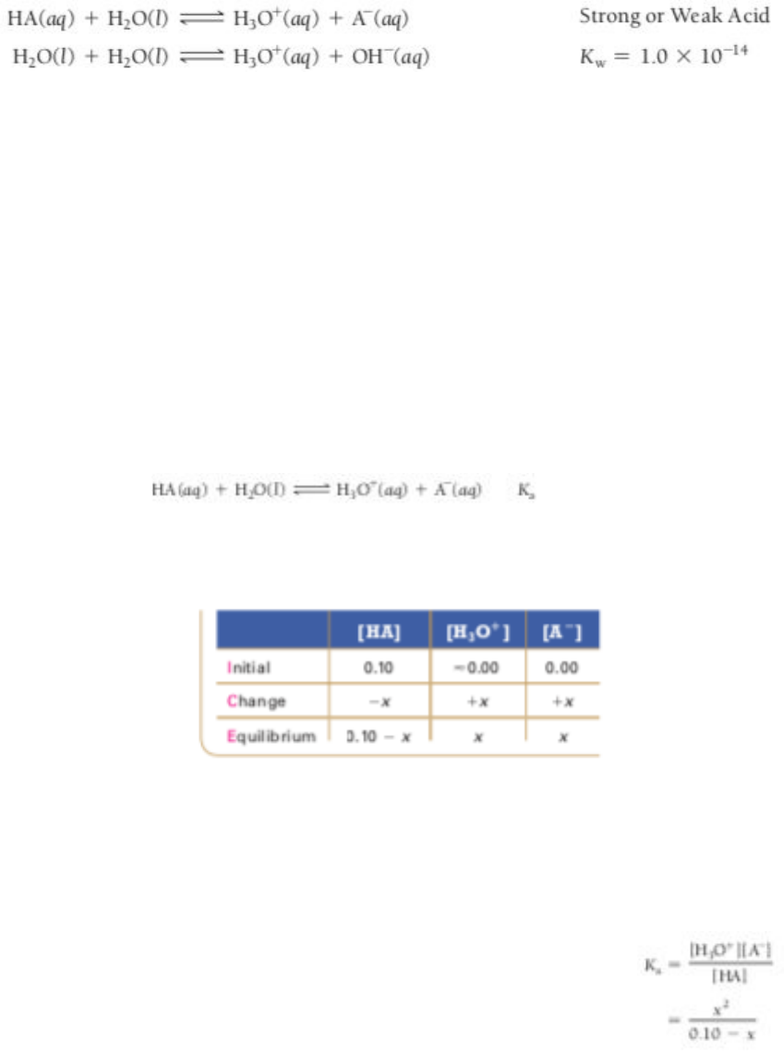Textbook Notes (270,000)
US (100,000)
Rutgers (1,000)
3:40 (200)
Chapter 16

# 01:160:162 Chapter Notes - Chapter 16: Rice Chart, Weak Base

Department
Chemistry
Course Code
01:160:162
Professor
Paul Kimmel
Chapter
16

This preview shows half of the first page. to view the full 3 pages of the document.Chemistry Textbook Notes 16.7-16.8
16.7 Finding the [H3O+] and pH of Strong and Weak Acid Solutions
- If we let HA be a strong or weak acid, the ionization reactions are:
- Except in extremely dilute acid solutions, the auto ionization of water contributes a negligibly
small amount of H3O+ compared to the ionization of the strong or weak acid
- Because strong acids, by definition, completely ionize in solution, and because we can ignore the
contribution of the auto ionization of water, the concentration of H3O+ in a strong acid
solution is equal to the concentration of the strong acid
- Determining the pH of a weak acid solution is more complicated because the concentration of
H3O+ is not equal to the concentration of the weak acid (because it only partially ionizes)
- Since we can ignore the contribution of the auto ionization of water, we only have too determine
concentration of H3O+ the
formed by the following equilibrium:
- And we can summarize the
initial conditions, the changes, and the
equilibrium conditions in the
following ICE table
- In the table, we list the initial H3O+ concentration as approximately zero
because of the negligibly small contribution of H3O+ due to the auto
ionization of water
- The variable x represents the amount of HA that ionizes
- We can use the equilibrium expression to set up an equation in which x is
the only variable
###### You're Reading a Preview

Unlock to view full version